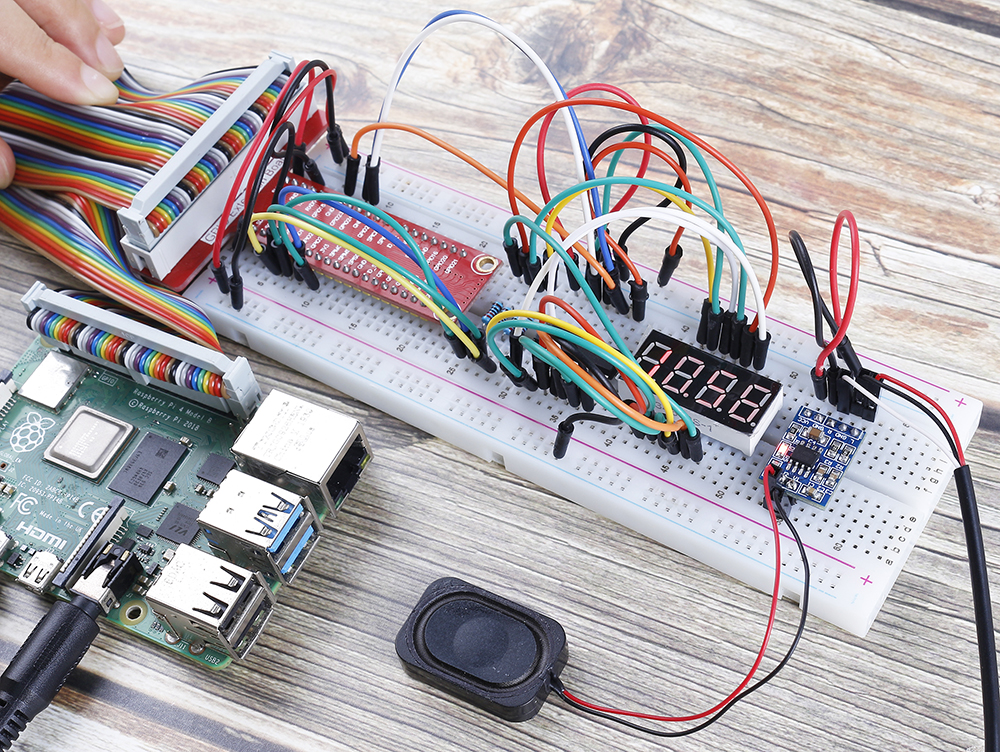# 4.1.3 Speech Clock¶

## Introduction¶

In this project, let’s make a voice clock with a speaker and a 4-digit 7-segment display. The 4-digit 7-segment display will display the time, and the speaker will broadcast the time every hour.

## Schematic Diagram¶

 T-Board Name physical wiringPi BCM GPIO17 Pin 11 0 17 GPIO27 Pin 13 2 27 GPIO22 Pin 15 3 22 SPIMOSI Pin 19 12 10 GPIO18 Pin 12 1 18 GPIO23 Pin 16 4 23 GPIO24 Pin 18 5 24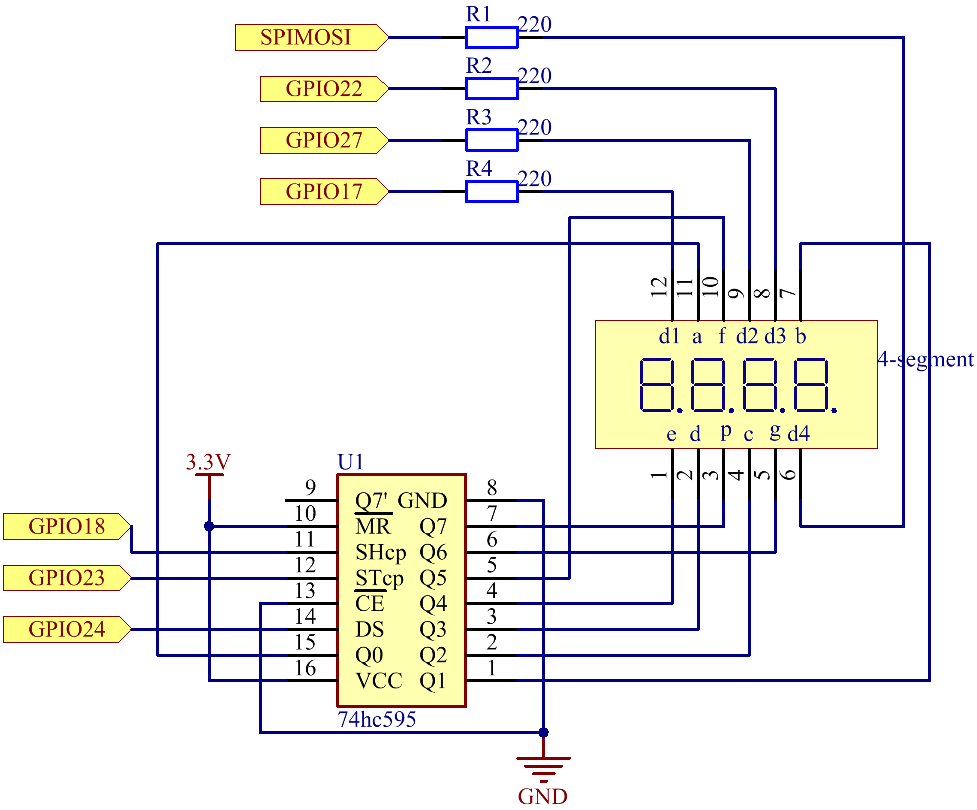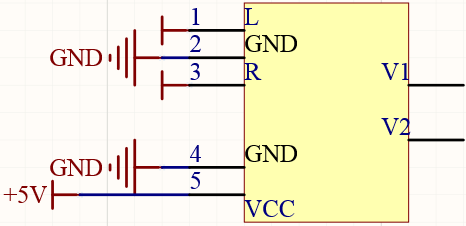## Experimental Procedures¶

Step 1: Build the circuit.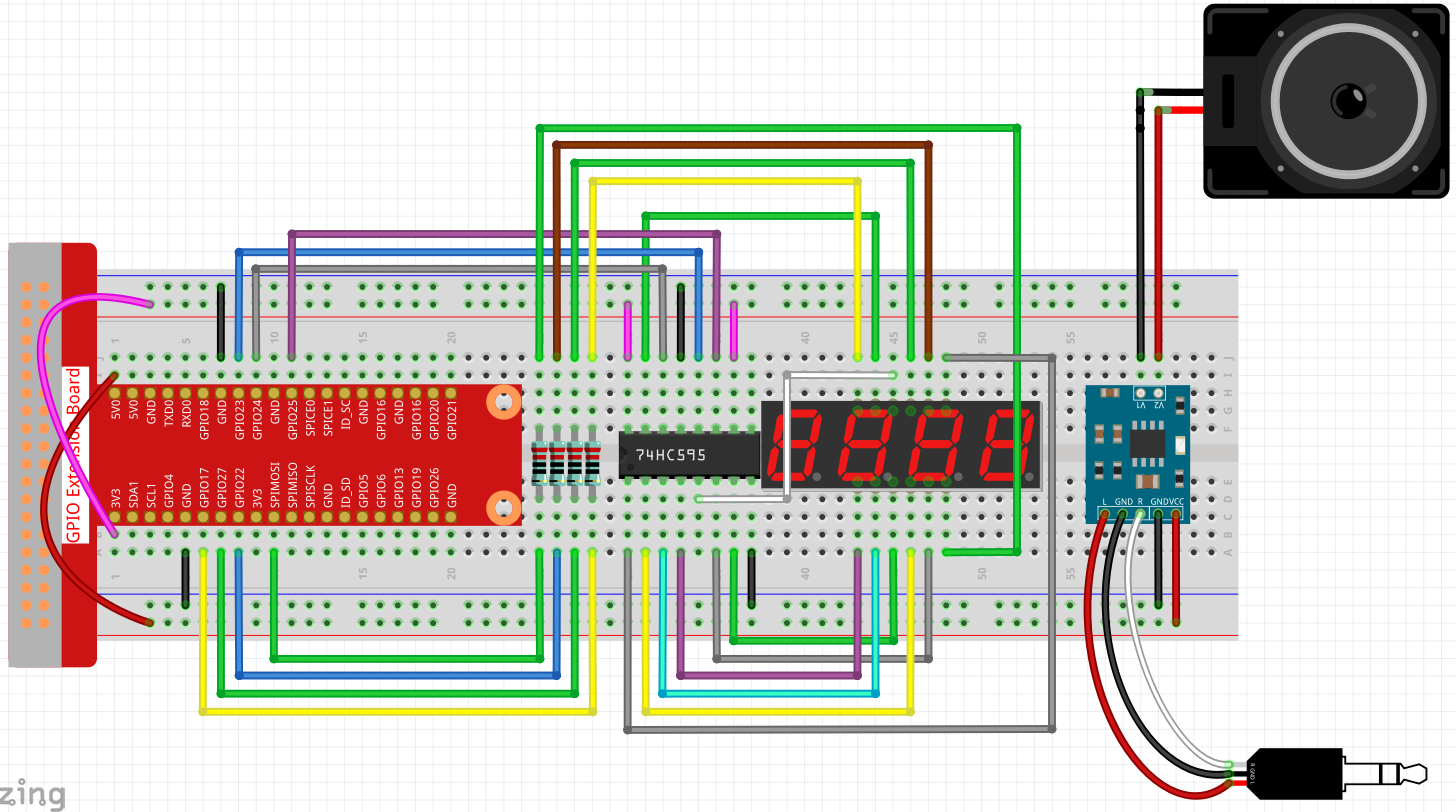Before this project, you need to make sure you complete 3.1.4 Text-to-speech.

Step 2: Use the command `date` to view the local time.

```date
```

If the local time is different from the real time, you need to use the following command to set the time zone.

```sudo dpkg-reconfigure tzdata
```

Choose your time zone.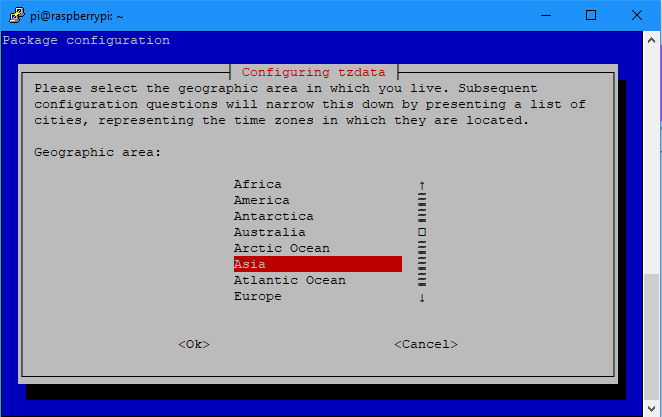Step 3: Get into the folder of the code.

```cd /home/pi/raphael-kit/python/
```

Step 3: Run.

```python3 4.1.3_SpeechClock.py
```

When the code is run, the 4-digit 7-segment will display the time and chime on every hour.

Code

Note

You can Modify/Reset/Copy/Run/Stop the code below. But before that, you need to go to source code path like `raphael-kit/python`. After modifying the code, you can run it directly to see the effect.

```import RPi.GPIO as GPIO
from tts import TTS
import time

tts = TTS(engine="espeak")
tts.lang('en-US')

SDI = 24
RCLK = 23
SRCLK = 18

placePin = (10, 22, 27, 17)
number = (0xc0, 0xf9, 0xa4, 0xb0, 0x99, 0x92, 0x82, 0xf8, 0x80, 0x90)

def setup():
GPIO.setmode(GPIO.BCM)
GPIO.setup(SDI, GPIO.OUT)
GPIO.setup(RCLK, GPIO.OUT)
GPIO.setup(SRCLK, GPIO.OUT)
for i in placePin:
GPIO.setup(i, GPIO.OUT)

def clearDisplay():
for i in range(8):
GPIO.output(SDI, 1)
GPIO.output(SRCLK, GPIO.HIGH)
GPIO.output(SRCLK, GPIO.LOW)
GPIO.output(RCLK, GPIO.HIGH)
GPIO.output(RCLK, GPIO.LOW)

def hc595_shift(data):
for i in range(8):
GPIO.output(SDI, 0x80 & (data << i))
GPIO.output(SRCLK, GPIO.HIGH)
GPIO.output(SRCLK, GPIO.LOW)
GPIO.output(RCLK, GPIO.HIGH)
GPIO.output(RCLK, GPIO.LOW)

def pickDigit(digit):
for i in placePin:
GPIO.output(i,GPIO.LOW)
GPIO.output(placePin[digit], GPIO.HIGH)

def loop():
status = 0
while True:
time.localtime(time.time())
hour = int(time.strftime('%H',time.localtime(time.time())))
minute = int(time.strftime('%M',time.localtime(time.time())))

clearDisplay()
pickDigit(0)
hc595_shift(number[minute % 10])

clearDisplay()
pickDigit(1)
hc595_shift(number[minute % 100//10])

clearDisplay()
pickDigit(2)
hc595_shift(number[hour % 10])

clearDisplay()
pickDigit(3)
hc595_shift(number[hour % 100//10])

if minute == 0 and status == 0:
tts.say('The time is now ' + str(hour) + ' hours and ' + str(minute) + ' minutes')
status = 1
elif minute != 0:
status = 0

def destroy():   # When "Ctrl+C" is pressed, the function is executed.
GPIO.cleanup()

if __name__ == '__main__':  # Program starting from here
setup()
try:
loop()
except KeyboardInterrupt:
destroy()
```

Code Explanation

```time.localtime(time.time())
hour = int(time.strftime('%H',time.localtime(time.time())))
minute = int(time.strftime('%M',time.localtime(time.time())))
```

Through the function `time.time()`, we can get the timestamp of the current time (the number of floating-point seconds that have passed since the 1970 epoch), and then use the time formatting method of the time module `(time.localtime(time.time()))` to process the current timestamp, so that we can format the timestamp as a local time.

The input result is:

`time.struct_time(tm_year=2021, tm_mon=5, tm_mday=28, tm_hour=13, tm_min=54, tm_sec=26, tm_wday=4, tm_yday=148, tm_isdst=0)`

Finally, we use the `time.strftime()` method to format the large string of information into what we want. If you want to get the current hour, you can get it through the function `time.strftime('%H',time.localtime(time.time()))` .

The output of the specified formatted string obtained by modifying the first parameter are listed below.

 %y Two-digit year representation(00-99) %Y Four-digit year representation(000-9999) %m month(01-12) %H Day of the month(0-31) %I Hours in a 24-hour clock(0-23) %M Hours in 12-hour clock(01-12) %y Minutes(00=59) %S second(00-59) %a Local simplified week name %A Full local week name %b Local simplified month name %B Local full month name %c Local corresponding date and time display %j Day of the year(001-366) %p The equivalent of local A.M. or P.M. %U Num of weeks of one year(00-53)starting with Sunday %w Week (0-6), starting with Sunday %W Num of weeks of one year(00-53)starting with Monday %x Local corresponding date representation %X Local corresponding time representation %Z The name of the current time zone

Note

The output of the `time.strftime()` method is all string variables. Before using it, remember to do a coercive type conversion.

```clearDisplay()
pickDigit(0)
hc595_shift(number[minute % 10])

clearDisplay()
pickDigit(1)
hc595_shift(number[minute % 100//10])

clearDisplay()
pickDigit(2)
hc595_shift(number[hour % 10])

clearDisplay()
pickDigit(3)
hc595_shift(number[hour % 100//10])
```

The tens digit of the hour is displayed on the first 7-segment digital display, and the ones digit is displayed on the second. Then the tens digit of the minutes is displayed on the third digital display, and the ones digit are displayed on the last.

```if minute == 0 and status == 0:
tts.say('The time is now ' + str(hour) + ' hours and ' + str(minute) + ' minutes')
status = 1
elif minute != 0:
status = 0
```

When the number of minutes is 0 (by hour), the Raspberry Pi will use TTS to announce the time for us.

## Phenomenon Picture¶